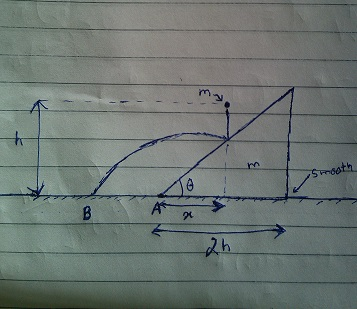# Challenges in mechanics by Ronak Agarwal (Part 2)A wedge of mass $m$ and angle $\theta$ is placed on a smooth ground. As shown in the diagram $A$ is a point on ground. A particle also having mass $m$ is dropped from a height $h$ at a horizontal distance $x$ from $A$. It finally touches the ground at point $B$. Find $\sqrt { 3 } AB$ in metres.

Details and Assumptions

$1)$Take $m=5Kg$, $h=2\sqrt { 2 }$ metre , $x=1$ metre.

$2)$ $tan(\theta)=\frac { 1 }{ \sqrt { 2 } }$

$3)$The collision of the ball with the wedge is elastic and the wedge is free to move

$4)$$g=9.8m/{ s }^{ 2 }$

###### Also try Solve for trajectory Again.
×

Problem Loading...

Note Loading...

Set Loading...